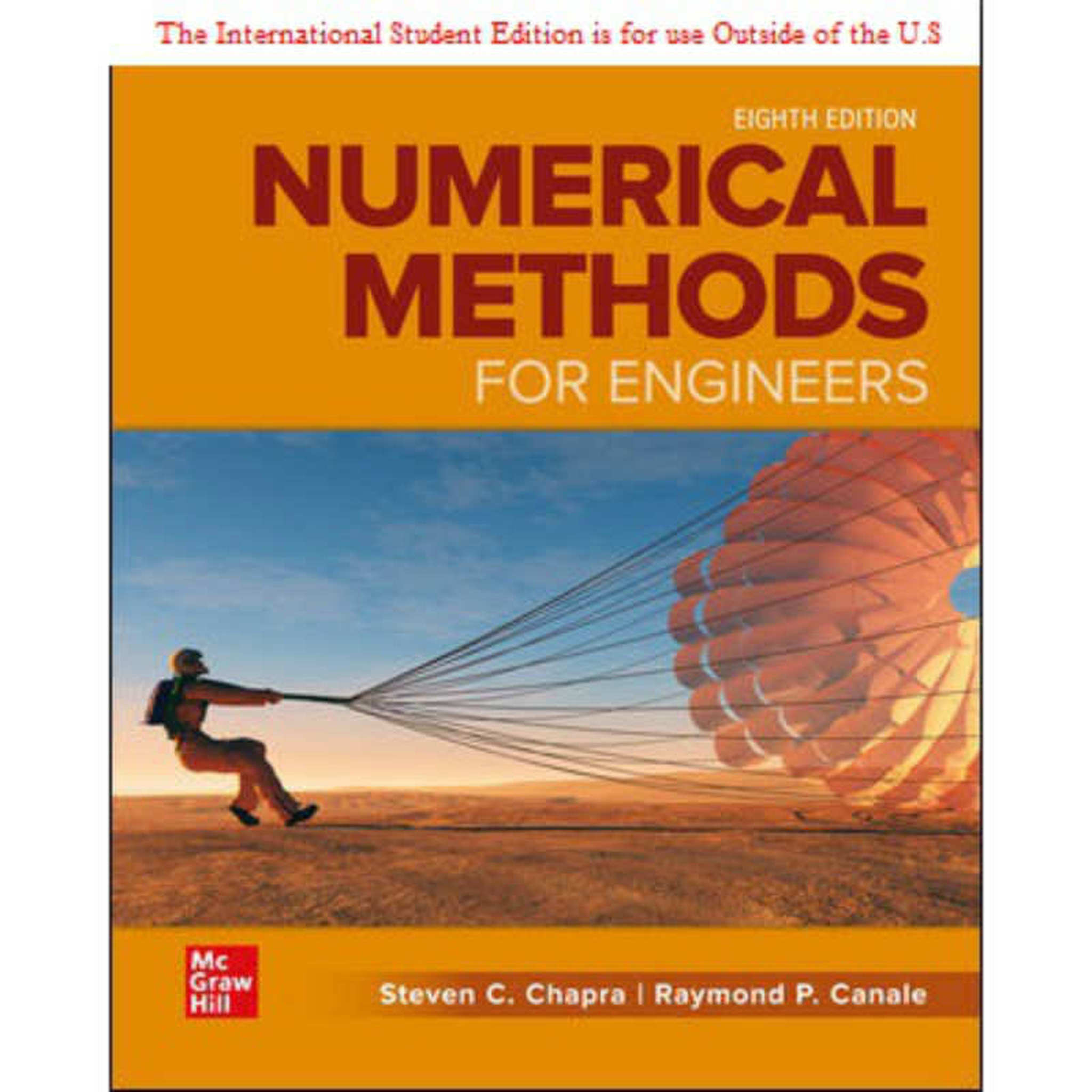# [U] NUMERICAL METHODS FOR ENGINEERS ISE 8E - STEVEN CHAPRA 9781260571387

RM 145.00

TITLE : NUMERICAL METHOD FOR ENGINEERS 8E - CHAPRA

ISBN13 : 9781260571387

PUBLISHER : MCGRAWHILL (2020)

EDITION : 8E ISE PAPERBACK

The eighth edition of Chapra and Canale's Numerical Methods for Engineers retains the instructional techniques that have made the text so successful. The book covers the standard numerical methods employed by both students and practicing engineers. Although relevant theory is covered, the primary emphasis is on how the methods are applied for engineering problem solving. Each part of the book includes a chapter devoted to case studies from the major engineering disciplines. Numerous new or revised end-of chapter problems and case studies are drawn from actual engineering practice. This edition also includes several new topics including a new formulation for cubic splines, Monte Carlo integration, and supplementary material on hyperbolic partial differential equations.

Part 1 - Modeling, Computers, and Error Analysis

1) Mathematical Modeling and Engineering Problem Solving
2) Programming and Software
3) Approximations and Round-Off Errors
4) Truncation Errors and the Taylor Series

Part 2 - Roots of Equations

5) Bracketing Methods
6) Open Methods
7) Roots of Polynomials
8) Case Studies: Roots of Equations

Part 3 - Linear Algebraic Equations

9) Gauss Elimination
10) LU Decomposition and Matrix Inversion
11) Special Matrices and Gauss-Seidel
12) Case Studies: Linear Algebraic Equations

Part 4 - Optimization

13) One-Dimensional Unconstrained Optimization
14) Multidimensional Unconstrained Optimization
15) Constrained Optimization
16) Case Studies: Optimization

Part 5 - Curve Fitting

17) Least-Squares Regression
18) Interpolation
19) Fourier Approximation
20) Case Studies: Curve Fitting

Part 6 - Numerical Differentiation and Integration

21) Newton-Cotes Integration Formulas
22) Integration of Equations
23) Numerical Differentiation
24) Case Studies: Numerical Integration and Differentiation

Part 7 - Ordinary Differential Equations

25) Runge-Kutta Methods
26) Stiffness and Multistep Methods
27) Boundary-Value and Eigenvalue Problems
28) Case Studies: Ordinary Differential Equations

Part 8 - Partial Differential Equations

29) Finite Difference: Elliptic Equations
30) Finite Difference: Parabolic Equations
31) Finite-Element Method
32) Case Studies: Partial Differential Equations

Appendix A - The Fourier Series
Appendix B - Getting Started with Matlab
Appendix C - Getting Starte dwith Mathcad